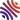## 11 publications1. WKB expansions for weakly well-posed hyperbolic boundary value problems in a strip: Time depending loss of derivatives (), In Journal of Hyperbolic Differential Equations, World Scientific Pub Co Pte Ltd, volume 18, . [hal]
2. Stability of finite difference schemes approximation for hyperbolic boundary value problems in an interval (), In Mathematics of Computation, American Mathematical Society (AMS), . [hal]
3. Lower exponential strong well-posedness of hyperbolic boundary value problems in a strip (), In Indiana Univ. Math. J., volume 69, . [hal-lmpa]
4. Weak well-posedness of hyperbolic boundary value problems in a strip: when instabilities do not reflect the geometry (), In Commun. Pure Appl. Anal., volume 19, . [hal]
5. WKB expansions for hyperbolic boundary value problems in a strip: selfinteraction meets strong well-posedness (), In J. Inst. Math. Jussieu, volume 19, . [hal]
6. Long-time homogenization and asymptotic ballistic transport of classical waves ( and ), In Ann. Sci. 'Ec. Norm. Sup'er. (4), volume 52, . [hal]
7. Semi-group stability of finite difference schemes in corner domains (), In Numer. Math. Theory Methods Appl., volume 11, . [hal]
8. Geometric optics expansions for hyperbolic corner problems II: From weak stability to violent instability (), In SIAM J. Math. Anal., volume 49, . [hal]
9. Geometric optics expansions for hyperbolic corner problems, I: self-interaction phenomenon (), In Anal. PDE, volume 9, . [hal]
10. Finite speed of propagation for mixed problems in the $WR$ class (), In Commun. Pure Appl. Anal., volume 13, . [hal]
11. Geometric optics expansions for linear hyperbolic boundary value problems and optimality of energy estimates for surface waves (), In Differential Integral Equations, volume 27, . [hal]